﻿ 多视图主动学习的多样性样本选择方法研究
«上一篇文章快速检索 高级检索

 智能系统学报2021, Vol. 16Issue (6): 1007-1014  DOI: 10.11992/tis.2020070370

### 引用本文CHEN Liwei, FANG He, ZHU Haifeng. Diversity sample selection method of multiview active learning classification[J]. CAAI Transactions on Intelligent Systems, 2021, 16(6): 1007-1014. DOI: 10.11992/tis.202007037.### 文章历史

Diversity sample selection method of multiview active learning classification
CHEN Liwei , FANG He , ZHU HaifengCollege of Information and Communication Engineering, Harbin Engineering University, Harbin 150001, China
Abstract: To remove the redundancy of selected samples in the multiview active learning classification of hyperspectral images and reduce the cost of manual marking, this paper proposes two methods for the selection of diverse samples in the multiview active learning classification. First, hyperspectral images are divided into superpixel segments, then samples belonging to different superpixel segments are added to the training set, and the remaining samples are put back into the candidate set. Second, the prediction labels of the samples from each view are compared, then the samples with different prediction labels are added into the training set, and the remaining samples are put back into the candidate set. In this study, the two methods are used to improve the sample selection method in the traditional multiview active learning classification, and experiments are conducted in two groups of hyperspectral image data. The results show that the accuracy of classification is unchanged, yet the number of training samples is greatly reduced after using the two methods.
Key words: hyperspectral image classification    multiview active learning    diversity    sample selection    superpixel    number of training samples    prediction labels    accuracy of classification

1 算法框架 1.1 MVAL的基本流程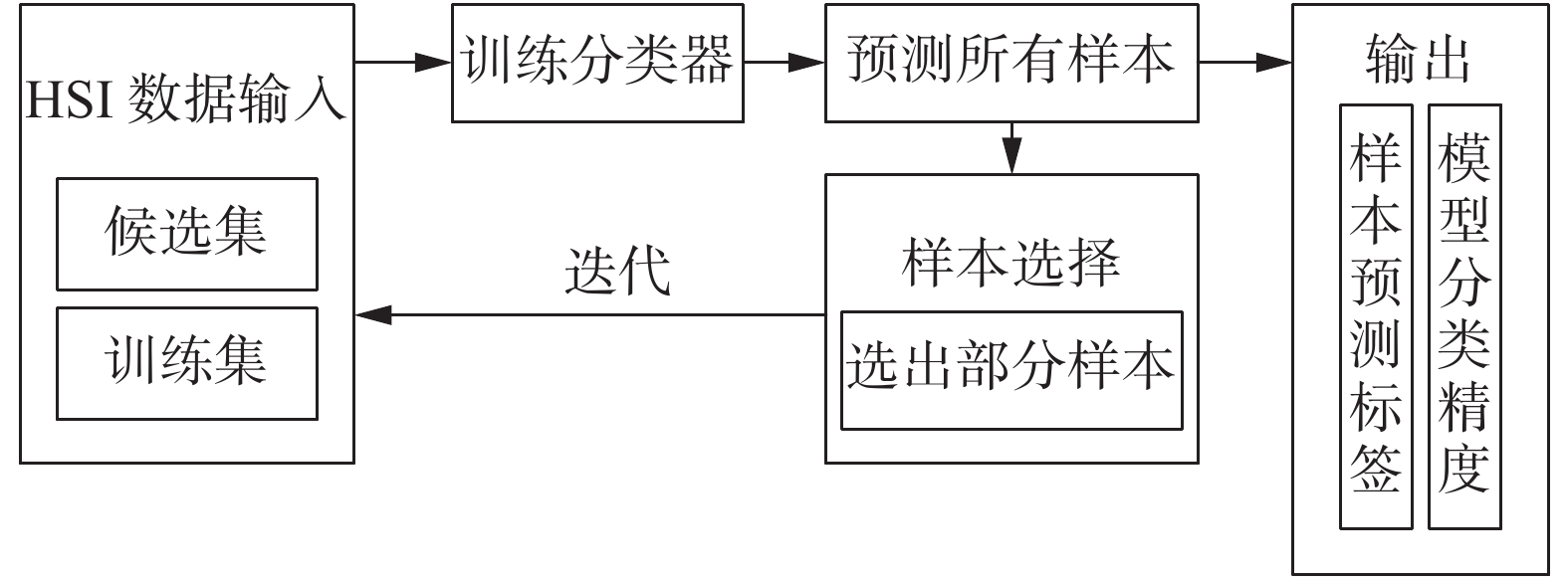Download: 图 1 基于MVAL的HSI分类的基本流程 Fig. 1 Basic flow chart of HSI classification based on MVAL
1.2 视图生成方法

 ${G_{\omega ,\varphi ,\theta }}(x,y,\lambda ) = g(x,y,\lambda )\exp \{ {\text{j}}(x{\omega _x} + y{\omega _y} + \lambda {\omega _\lambda })\}$

 \begin{aligned} \varphi_{\rm{FR}}=\frac{1}{B}\frac{2}{{r(r - 1)}}{\sum\limits_b^B}{\sum\limits_{i,j \in \{ 1,2, \cdots ,r\} } } {\sum\limits_{x \in {D_s}}}\quad\\ {\frac{{({{\boldsymbol{\mu}} _i} - {{\boldsymbol{\mu}} _j}){{({{\boldsymbol{\mu}} _i} - {{\boldsymbol{\mu}} _j})}^{\rm T}}}}{{({{\boldsymbol{\mu}} _i} - x){{({{\boldsymbol{\mu}} _i} - x)}^{\rm T}} + ({{\boldsymbol{\mu}} _j} - x){{({{\boldsymbol{\mu}} _j} - x)}^{\rm T}}}}}\end{aligned}

1.3 传统的AMD查询策略

 ${Q_{{\text{AMD}}}} = \mathop {\arg \max }\limits_{{x_i} \in {D_C}} |{L_k}({x_i}){|_{{\text{count}}}} \text{，} k = 1,2, \cdots ,K$

2 MVAL样本选择方法的多样性选择策略

2.1 基于超像素分割的多样性选择策略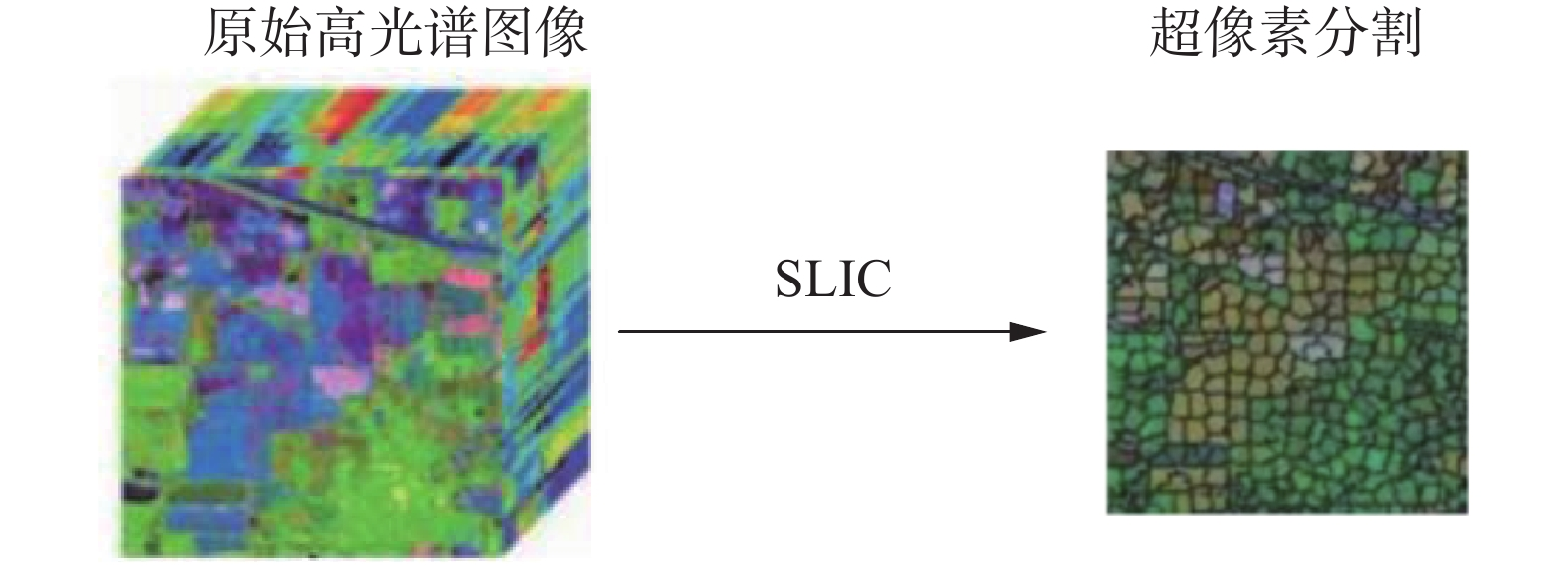Download: 图 2 高光谱图像的SLIC超像素分割图 Fig. 2 SLIC superpixel segmentation schematic of hyperspectral images

 ${L_i} = L({x_i}), \quad {L_i} \in [1,k]$

 ${{\boldsymbol{X}}_{{\text{SLIC}}}} = {\text{unique}}\left[ {L({x_1})\;L({x_2})\; \cdots \;L({x_m})} \right]$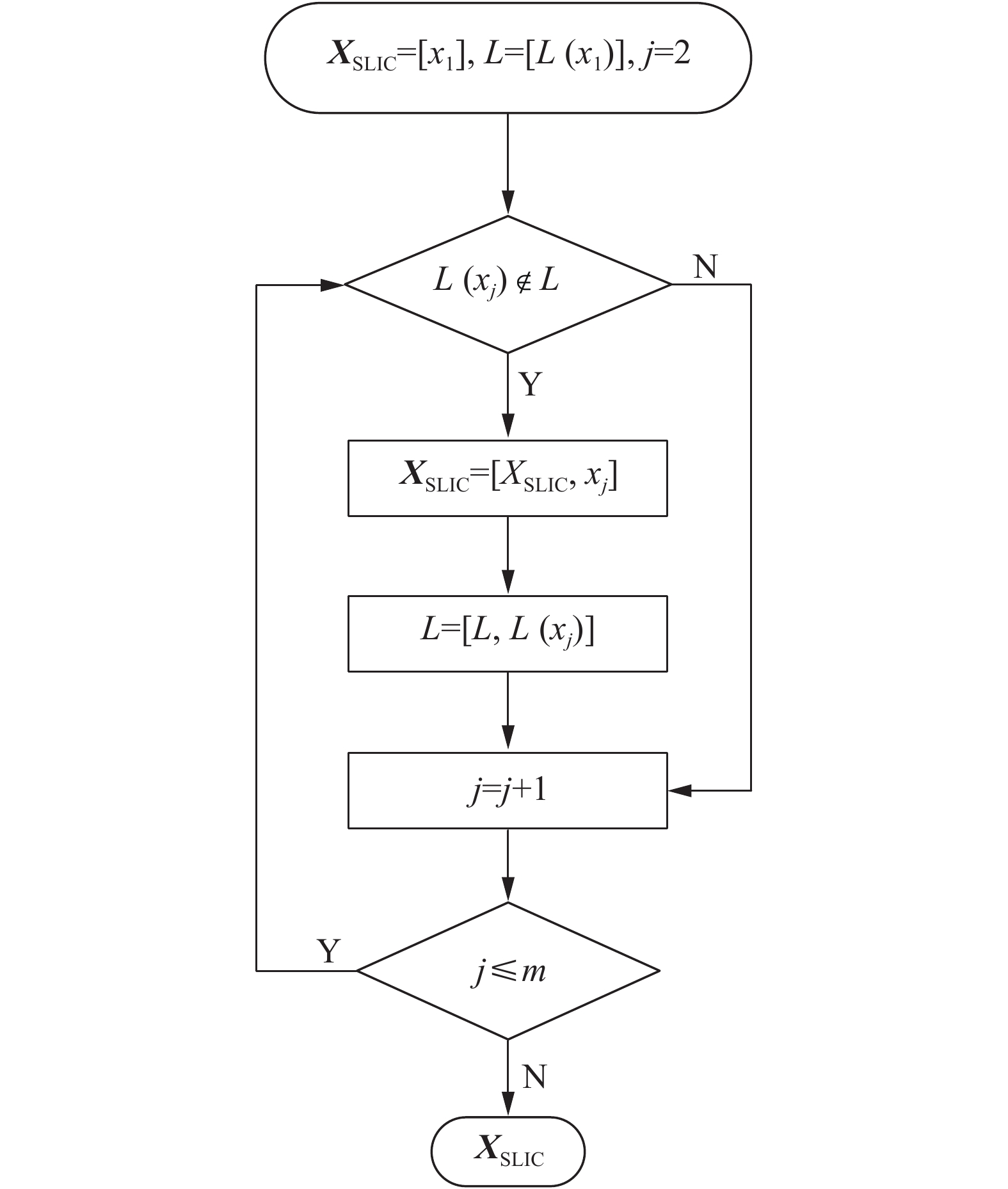Download: 图 3 unique函数的流程 Fig. 3 Flow of unique function

2.2 基于预测标签一致性的多样性选择策略

 $D = \sum\limits_{k = 1}^K | {L_k}({x_i}),{L_k}({x_j}){|_{{\text{equal}}}}$
 $|a,b{|_{{\text{equal}}}} = \left\{ \begin{gathered} 1,\quad a = b \hfill \\ 0, \quad a \ne b \hfill \\ \end{gathered} \right.$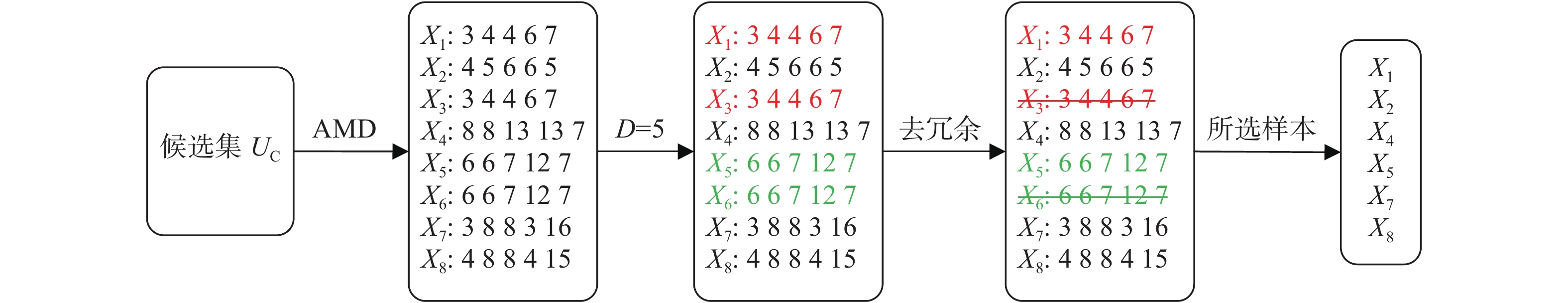Download: 图 4 基于预测标签一致性的多样性样本选择方法示意 Fig. 4 Graphical representation of a diversity sampling method based on predictive label consistency
3 实验结果与讨论 3.1 实验数据集

3.2 分类性能评价指标

OA方法通过混淆矩阵判别总体分类精度，具体表达式为

 ${\text{OA = }}\frac{{\text{1}}}{N}\sum\limits_{i = 1}^n {{m_i}}$

AA表示在每个类别中分类正确的样本所占的比重。如果各类别样本数量相同，则平均分类精度AA与总体分类精度OA相同。AA的表达式为

 ${\text{AA = }}\frac{{\text{1}}}{N}\sum\limits_{i = 1}^n {\rm{CA}}$

Kappa系数用来表示分类图与真实图像的一致性。Kappa系数的具体表达式为

 ${\text{Kappa}} = \dfrac{{N \times \displaystyle\sum\limits_{i = 1}^n {{m_i}} - \displaystyle\sum\limits_{i = 1}^n {{N_i} \times {m_i}} }}{{{N^2} - \displaystyle\sum\limits_{i = 1}^n {{N_i} \times {m_i}} }}$
3.3 实验结果对比表 1 Indian Pines数据集的AMD+A与AMD的实验结果 Tab.1 Experimental results of AMD+A and AMD in the Indian Pines dataset表 2 Salinas数据集的AMD+A与AMD的实验结果 Tab.2 Experimental results of AMD+A and AMD in the Salinas dataset表 3 AMD+A与AMD实验的最终结果 Tab.3 Final results of the AMD+A and AMD experiments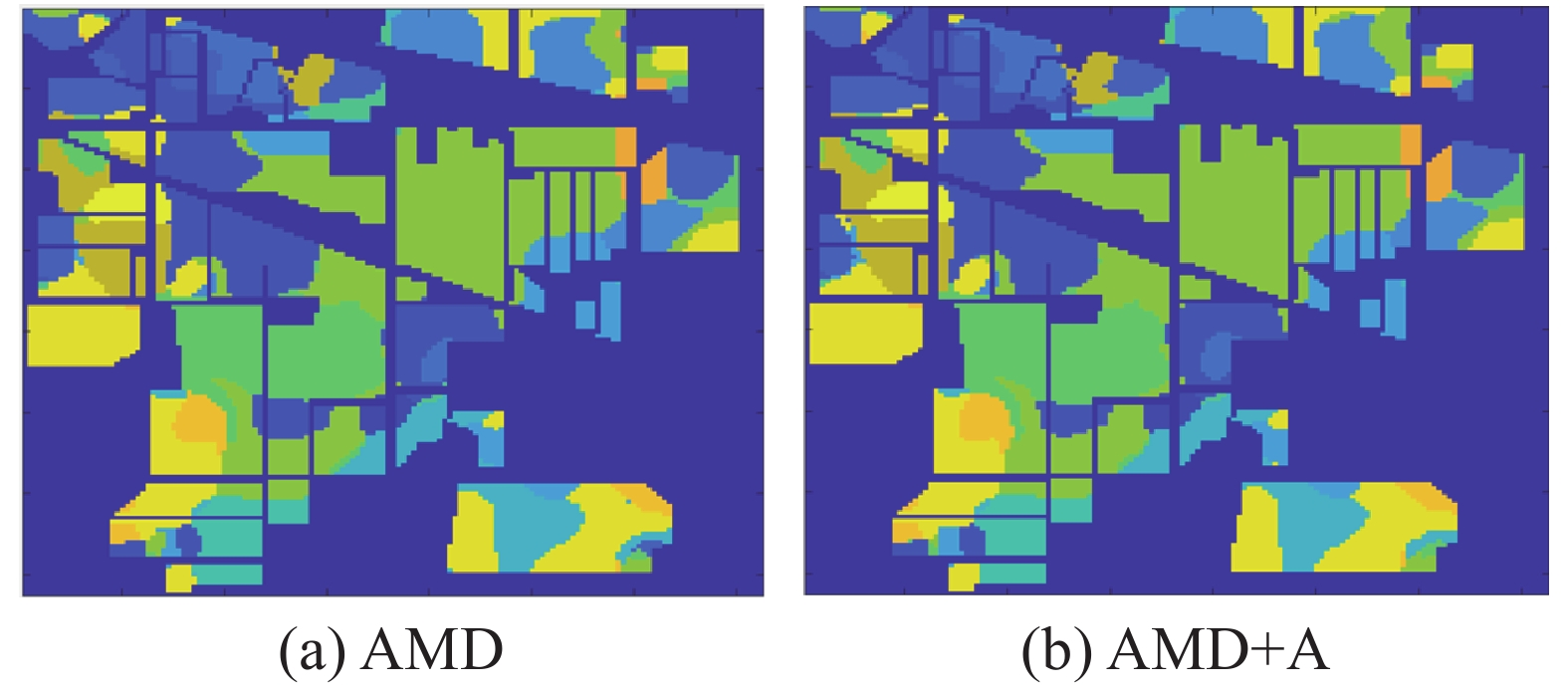Download: 图 5 用方法A改进前后的实验结果分析(Indian Pines) Fig. 5 Comparison of experimental results before and after improvement with method A (Indian Pines)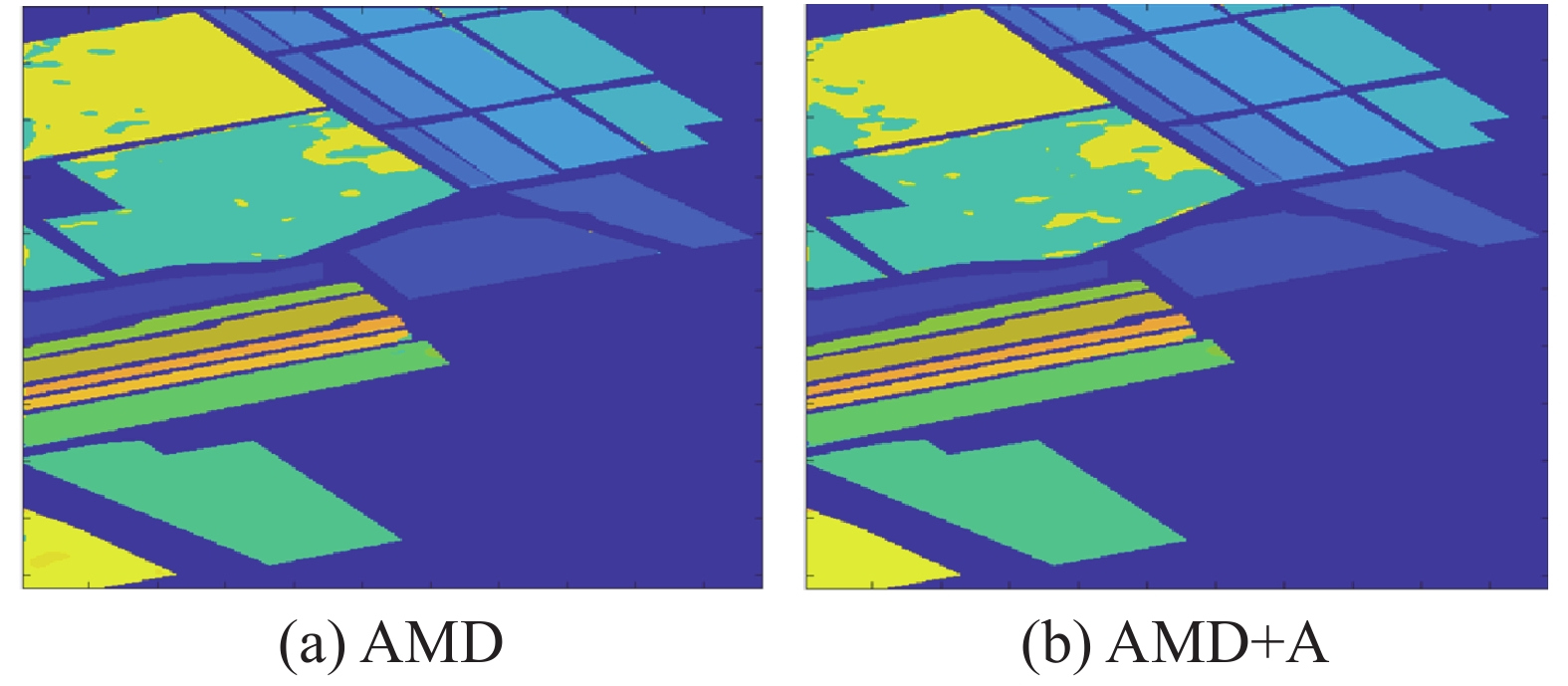Download: 图 6 用方法A改进前后的实验结果分析(Salinas) Fig. 6 Comparison of experimental results before and after improvement with method A (Salinas)表 4 Indian Pines数据集的AMD+B与AMD的实验结果 Tab.4 Experimental results of AMD+B and AMD in the Indian Pines dataset表 5 Salinas数据集的AMD+B与AMD的实验结果 Tab.5 Experimental results of AMD+B and AMD in the Salinas dataset表 6 AMD+B与AMD实验的最终结果 Tab.6 Final results of AMD+B and AMD experiments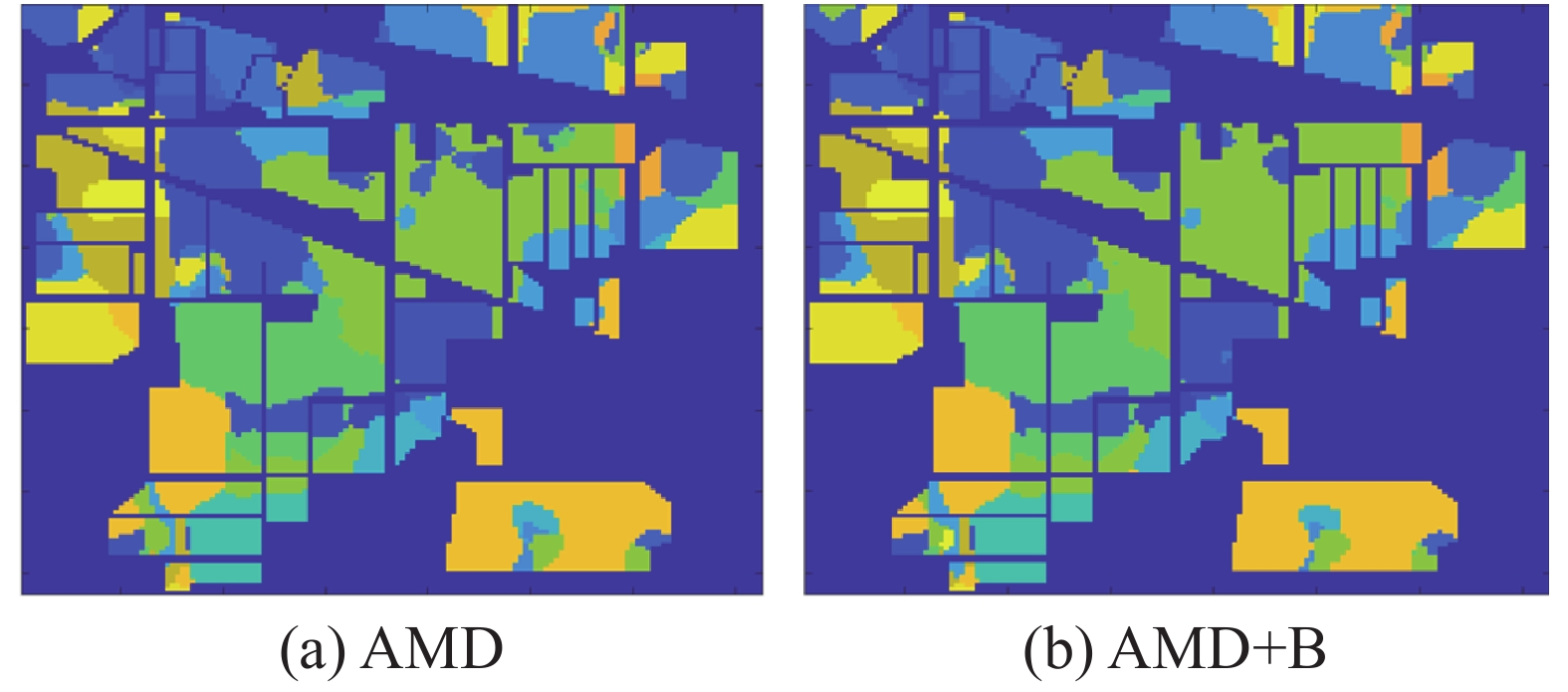Download: 图 7 用方法B改进前后的实验结果分析(Indian Pines) Fig. 7 Comparison of experimental results before and after improvement with method B (Indian Pines)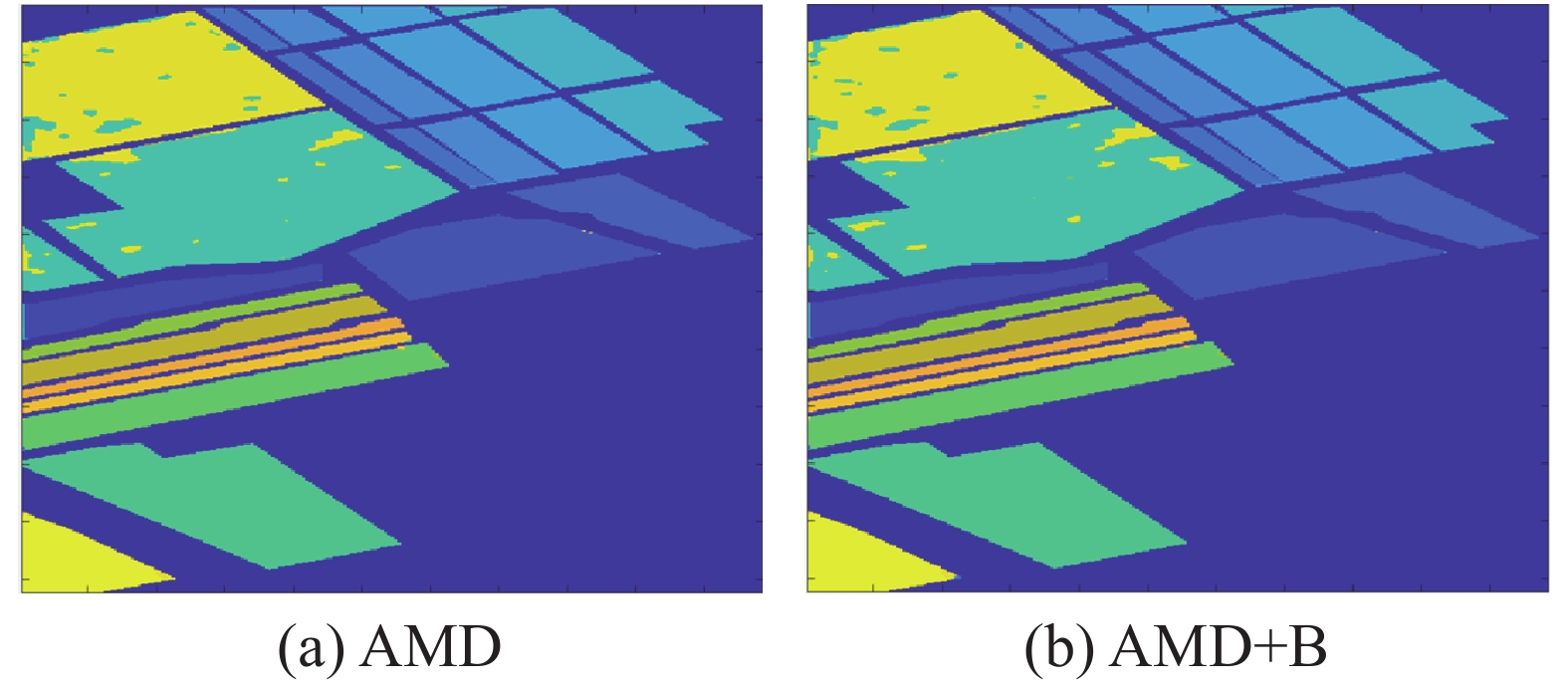Download: 图 8 用方法B改进前后的实验结果分析(Salinas) Fig. 8 Comparison of experimental results before and after improvement with method B (Salinas)

4 结束语

  LU Xiaohui, XIA Zhengyan, QU Fangfang, et al. Identification of authenticity, quality and origin of saffron using hyperspectral imaging and multivariate spectral analysis[J]. Spectroscopy letters, 2020, 53(2): 76-85. DOI:10.1080/00387010.2019.1693403 (0)  张晓玲, 张培强, 沈兰荪. 基于信息量失真测度的VQ及在高光谱图像无损压缩中的应用[J]. 遥感学报, 2004, 8(5): 414-418. ZHANG Xiaoling, ZHANG Peiqiang, SHEN Lansun. A VQ based on information distortion measure and its application to lossless compression of hyperspectral image[J]. Journal of remote sensing, 2004, 8(5): 414-418. (0)  WANG Meng, HUA Xiansheng. Active learning in multimedia annotation and retrieval: a survey[J]. ACM transactions on intelligent systems and technology, 2011, 2(2): 10. (0)  TU Bing, KUANG Wenlan, ZHAO Guangzhe, et al. Hyperspectral image classification by combining local binary pattern and joint sparse representation[J]. International journal of remote sensing, 2019, 40(24): 9484-9500. DOI:10.1080/01431161.2019.1633699 (0)  YANG Jihai, LI Shijun, XU Wenning. Active learning for visual image classification method based on transfer learning[J]. IEEE access, 2017, 6: 187-198. (0)  LIN Jianzhe, ZHAO Liang, LI Shuying, et al. Active-learning-incorporated deep transfer learning for hyperspectral image classification[J]. IEEE journal of selected topics in applied earth observations and remote sensing, 2018, 11(11): 4048-4062. DOI:10.1109/JSTARS.2018.2874225 (0)  赵忠明, 高连如, 陈东, 等. 卫星遥感及图像处理平台发展[J]. 中国图象图形学报, 2019, 24(12): 2098-2110. ZHAO Zhongming, GAO Lianru, CHEN Dong, et al. Development of satellite remote sensing and image processing platform[J]. Journal of image and graphics, 2019, 24(12): 2098-2110. DOI:10.11834/jig.190450 (0)  程圆娥, 周绍光, 袁春琦, 等. 基于主动深度学习的高光谱影像分类[J]. 计算机工程与应用, 2017, 53(17): 192-196, 248. CHENG Yuan’e, ZHOU Shaoguang, YUAN Chunqi, et al. Hyperspectral image classification based on active deep learning[J]. Computer engineering and applications, 2017, 53(17): 192-196, 248. DOI:10.3778/j.issn.1002-8331.1611-0174 (0)  吴超. 高光谱图像处理若干关键技术研究[D]. 南京: 南京航空航天大学, 2012. WU Chao. Research on some key technologies of hyperspectral image processing[D]. Nanjing: Nanjing University of Aeronautics and Astronautics, 2012. (0)  JAMSHIDPOUR N, SAFARI A, HOMAYOUNI S. Multiview active learning optimization based on genetic algorithm and Gaussian mixture models for hyperspectral data[J]. IEEE geoscience and remote sensing letters, 2020, 17(1): 172-176. DOI:10.1109/LGRS.2019.2914858 (0)  DI Wei, CRAWFORD M M. View generation for multiview maximum disagreement based active learning for hyperspectral image classification[J]. IEEE transactions on geoscience and remote sensing, 2012, 50(5): 1942-1954. DOI:10.1109/TGRS.2011.2168566 (0)  DI Wei, CRAWFORD M M. Multi-view adaptive disagreement based active learning for hyperspectral image classification[C]//2010 IEEE International Geoscience and Remote Sensing Symposium. Honolulu, USA, 2010: 1374−1377. (0)  甘继生. 基于广义组合核的高光谱图像GPU并行分类[D]. 南京: 南京理工大学, 2018. GAN Jisheng. Hyperspectral Image GPU parallel classification based on generalized composite cores[D]. Nanjing: Nanjing University of Science and Technology, 2012. (0)  HU Jie, HE Zhi, LI Jun, et al. 3D-gabor inspired multiview active learning for spectral-spatial hyperspectral image classification[J]. Remote sensing, 2018, 10(7): 1-24. (0)  XU Xiang, LI Jun, LI Shutao. Multiview intensity-based active learning for hyperspectral image classification[J]. IEEE transactions on geoscience and remote sensing, 2018, 56(2): 669-680. DOI:10.1109/TGRS.2017.2752738 (0)  魏飞鸣, 李小文, 顾行发, 等. 基于形状参数的遥感图像“同谱异物”目标区分[C]//第十四届全国图象图形学学术会议论文集. 福州, 中国, 2008: 477−481. WEI Feiming, LI Xiaowen, GU Xingfa, et al. Shape-based classification of “Spectral Similar” objects in remote sensing image processing[C]//Proceedings of the 14th National Conference on Image Graphics. Fuzhou, China, 2008: 477−481. (0)  王立国, 魏芳洁. 结合APO算法的高光谱图像波段选择[J]. 哈尔滨工业大学学报, 2013, 45(9): 100-106. WANG Liguo, WEI Fangjie. Artificial physics optimization algorithm combined band selection for hyperspectral imagery[J]. Journal of Harbin Institute of Technology, 2013, 45(9): 100-106. (0)  王阳. 基于超像素的图像分割算法研究[D]. 兰州: 兰州理工大学, 2018: 1−57. WANG Yang. Research on image segmentation algorithm based on superpixel[D]. Lanzhou: Lanzhou University of Technology, 2018: 1−57. (0)  LIU Peng, HUANG Jiwei, ZHANG Shiwu, et al. Multiview hyperspectral topography of tissue structural and functional characteristics[J]. Journal of biomedical optics, 2016, 21(1): 1-12. DOI:10.1117/1.JBO.21.1.015008 (0)  SUN Shiliang. A survey of multi-view machine learning[J]. Neural computing and applications, 2013, 23(7/8): 2031-2038. (0)  ZHAO Jing, XIE Xijiong, XU Xin, et al. Multi-view learning overview: recent progress and new challenges[J]. Information fusion, 2017, 38: 43-54. DOI:10.1016/j.inffus.2017.02.007 (0)  HE Lin, LI Jun, PLAZA A, et al. Discriminative low-rank Gabor filtering for spectral–spatial hyperspectral image classification[J]. IEEE transactions on geoscience and remote sensing, 2017, 55(3): 1381-1395. DOI:10.1109/TGRS.2016.2623742 (0)  段无悔. 基于超像素的高光谱图像分类算法研究[D]. 长沙: 湖南大学, 2015: 1−64. DUAN Wuhui. Researches on hyperspectral image classification algorithms based on superpixel[D]. Changsha: Hunan University, 2015: 1−64. (0)  PATRA S, BHARDWAJ K, BRUZZONE L. A spectral-spatial multicriteria active learning technique for hyperspectral image classification[J]. IEEE journal of selected topics in applied earth observations and remote sensing, 2017, 10(12): 5213-5227. DOI:10.1109/JSTARS.2017.2747600 (0)  BIOUCASDIAS B J, FIGUEIREDO M. Logistic regression via variable splitting and augmented lagrangian tools[R]. Instituto Superior Técnico, TULisbon, 2009. (0)